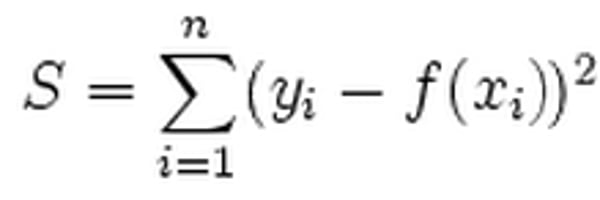Related Tags

l2
norm
distance
machine learning

# L2 norm in PythonEducative Answers Team

The $L_2$ norm loss function, also known as the least squares error (LSE), is used to minimize the sum of the square of differences between the target value, $Y_i$, and the estimated value, $f(x_i)$

The mathematical representation of $L_2$-norm is:As an error function, $L_2$-norm is less robust to outliers than the $L_1$-norm. An outlier causes the error value to increase to a much larger number because the difference in the actual and predicted value gets squared.

However, $L_2$-norm always provides one stable solution (unlike $L_1$-norm).

The $L_1$-norm loss function is known as the least absolute error (LAE) and ​is used to minimize the sum of absolute differences between the target value, $Y_i$, and the estimated values, $f(x_i)$.

## Code

The code to implement the $L_2$-norm is given below:

import numpy as np
actual_value = np.array([1, 2, 3])
predicted_value = np.array([1.1, 2.1, 5 ])

# take square of differences and sum them
l2 = np.sum(np.power((actual_value-predicted_value),2))
print(l2)

RELATED TAGS

l2
norm
distance
machine learning